Crab Walk Relay Race

Mr. Fair, the physical education teacher, is forming three crab walk relay teams. There must be four students on each relay team. To determine the teams, Mr. Fair uses the students' crab walk times from the last physical education class. The students are timed to the nearest tenth of a second.

 Student Time in Seconds Harry 12.2 Sally 10.3 Joseph 8.9 Amy 8.7 Ryan 9.9 Josh 11.4 Meredith 10.5 Anna 9.1 Brooke 10.9 Travis 11.1 Greg 11.9 Emily 11

Mr. Fair wants each of the three relay teams to be as equally matched in total time as possible. Mr. Fair writes the three teams on a piece of paper. What four students could Mr. Fair put on each team? Mr. Fair decides to write the less than, greater than, or equal symbols on his paper to compare the three teams. What could be on Mr. Fair's paper? Show all your mathematical thinking.

Assessment

Plan

Formal Mathematical Language and Symbolic Notation

A student may independently select a printed number line, number chart, ten frames, graph paper, etc. as they work on a task.

Solutions tabs

Expert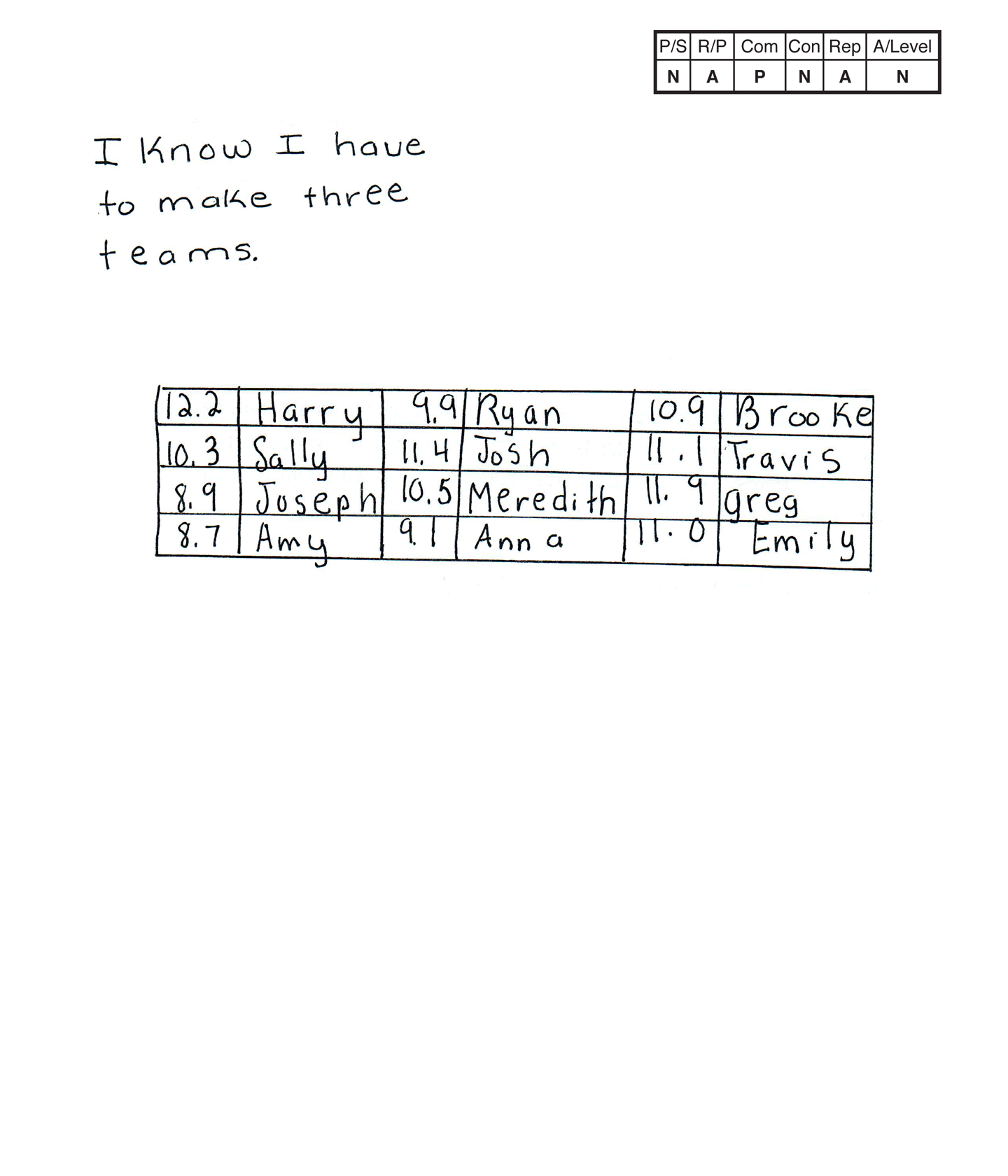This student does not meet the standard.

Scoring Rationale

Novice

The student's strategy of making a chart with the students listed in the order they appear on Mr. Fair's practice times chart does not work to solve the first part of the task. The student does not answer the second part of the task. The student does not apply the less than, greater than, or equal symbols to compare the three teams.

Apprentice

The student demonstrates understanding that Mr. Fair requires three teams of four students. The student does not demonstrate understanding that the total seconds for each team needs to be calculated to determine if the teams are equally matched. The student does not indicate understanding of applying the greater than, less than, or equal sign to compare the three teams.

Practitioner

The student correctly uses the mathematical notation 12.2, 10.3, 8.9, 8.7, 9.9, 11.4, 10.5, 9.1, 10.9, 11.1, 11.9, 11.0 from the task.

Novice

The student does not make a mathematically relevant observation.

Apprentice

The student attempts to construct a chart to support their reasoning. The chart does not have labels for each of the six columns and the seconds unit is not applied.

Novice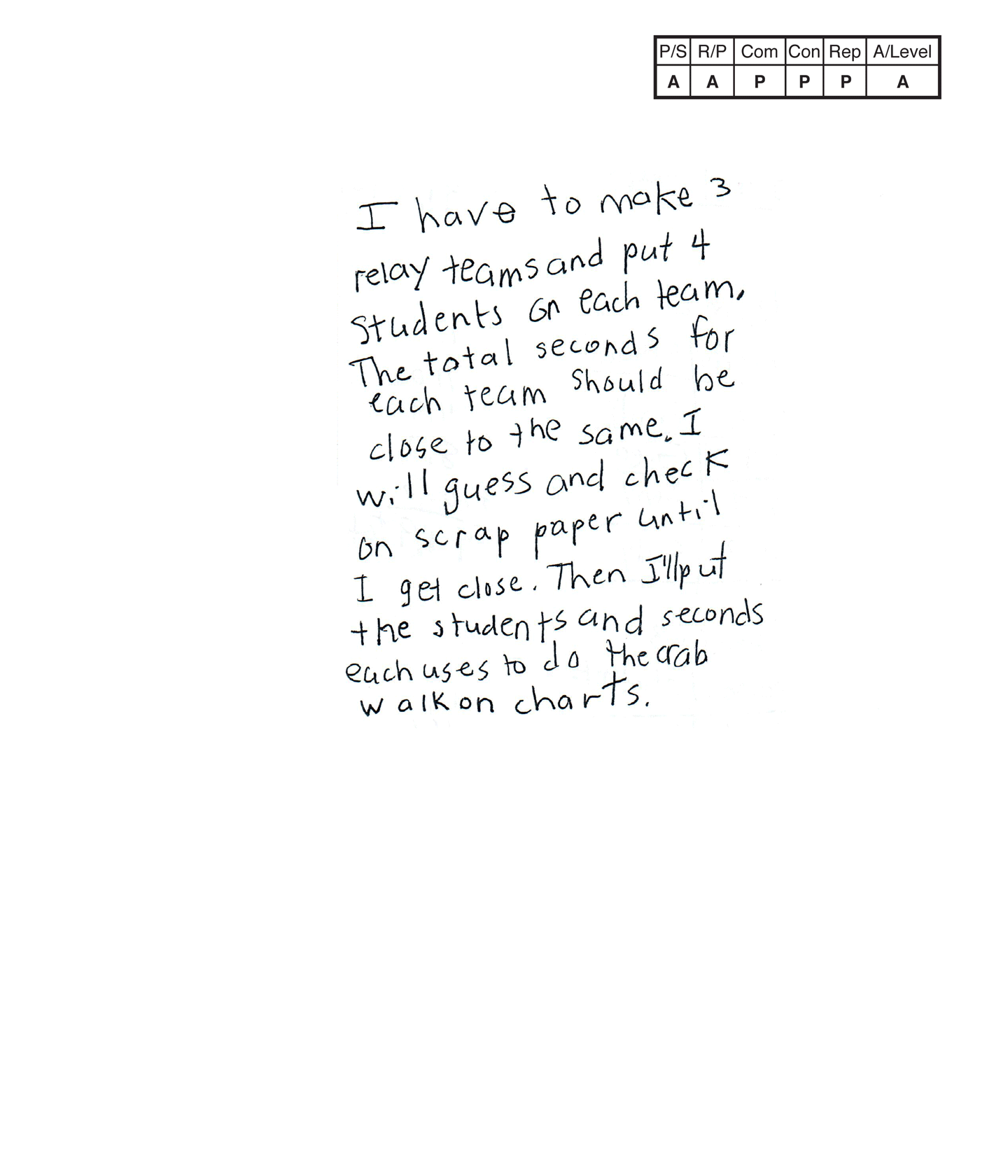,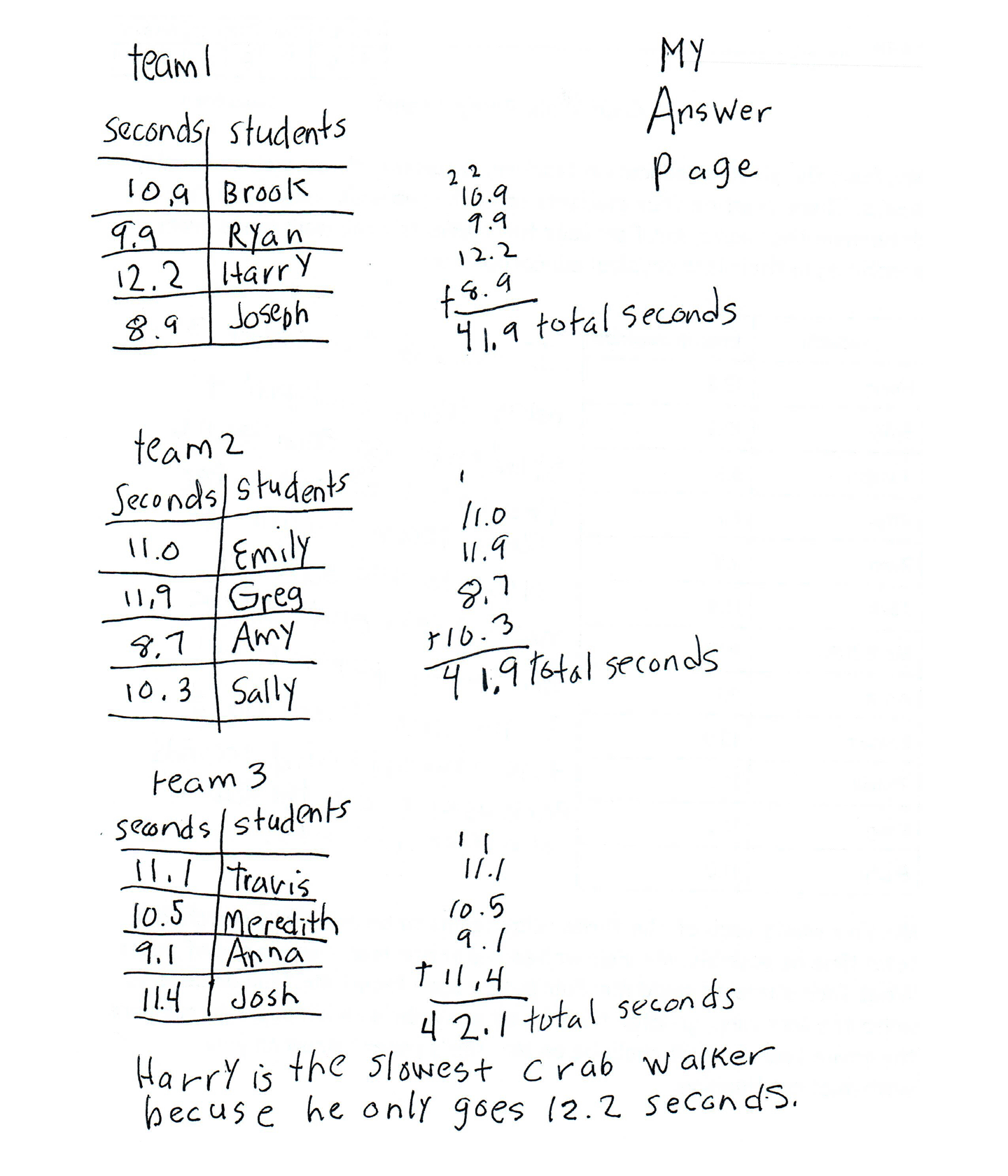This student does not meet the standard.

Scoring Rationale

Apprentice

The student's strategy of creating a table of three teams of students and calculating the total seconds per team to determine if they are equally matched works to solve the first part of the task. The student's tables on their "My Answer Page," correctly represent three equally matched teams. The student does not address the second part of the task.

Apprentice

The student demonstrates understanding that Mr. Fair requires three possible teams of four students that are as equally matched as possible. The student does not show correct reasoning for the second part of the task.

Practitioner

The student correctly uses the mathematical term seconds from the task. The student also correctly uses the terms charts, total. The student correctly uses the notation 10.9, 9.9, 12.2, 8.9, 11.0, 11.9, 8.7, 10.3, 11.1, 10.5, 9.1, 11.4 from the task. The student also correctly uses the notation 41.9, 42.1.

Practitioner

The student makes the mathematically relevant observation, "Harry is the slowest crab walker becuse he only goes 12.2 seconds."

Practitioner

The student's three tables are appropriate to the task and accurate. Each team is labeled, the columns are correctly labeled and all entered data is correct.

Apprentice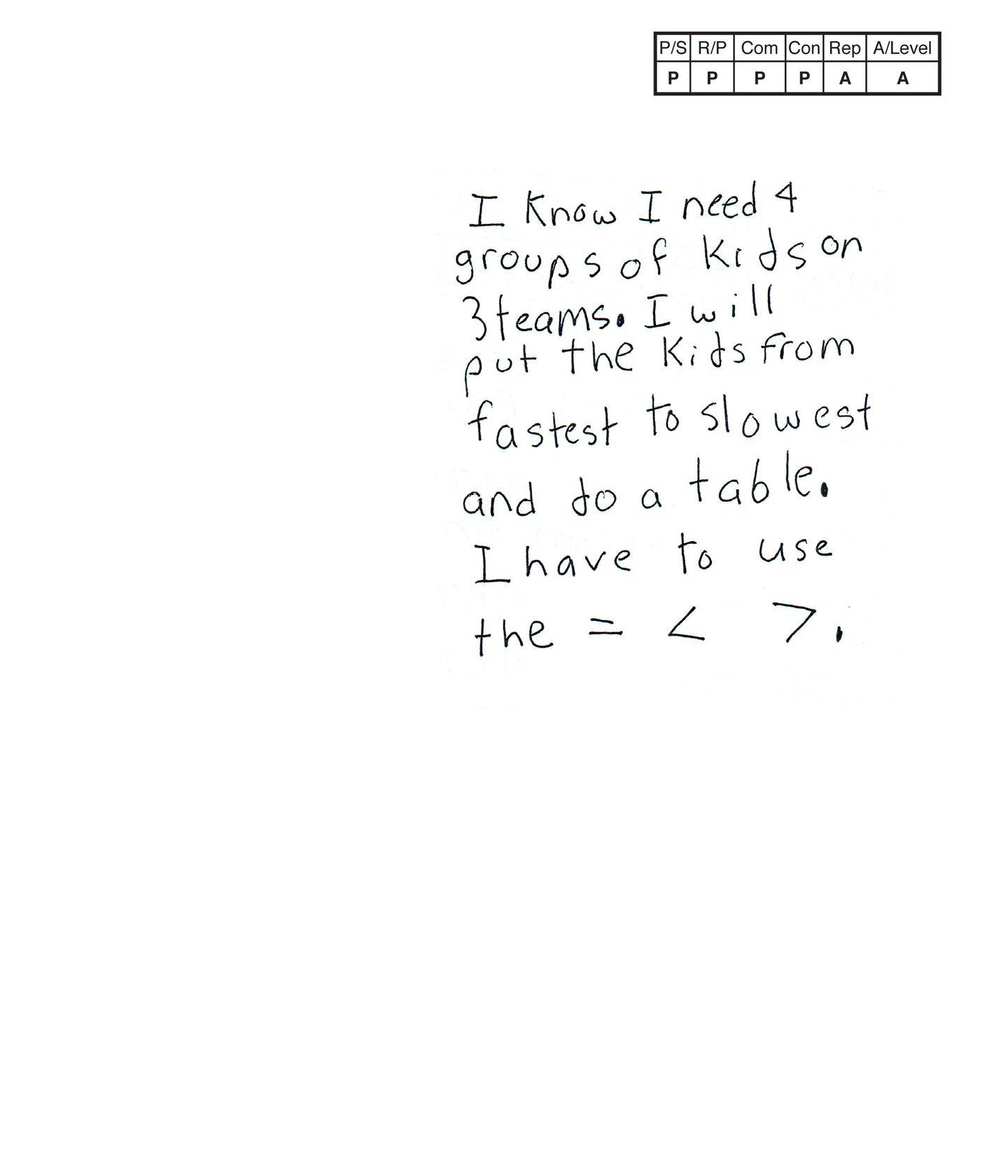,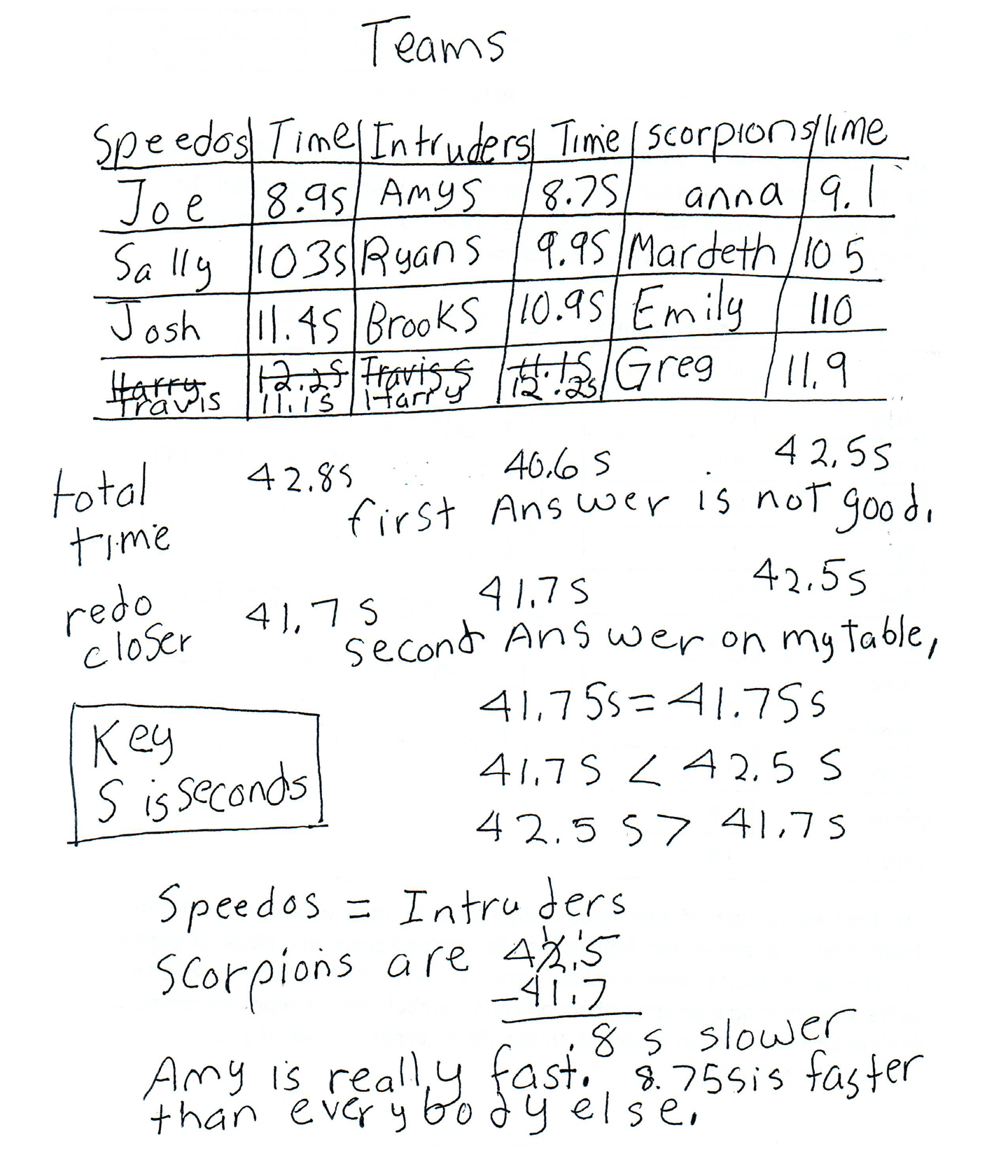This student does not meet the standard.

Scoring Rationale

Practitioner

The student's strategy of creating a table of three teams of students and calculating the total seconds per team to determine if they are equally matched works to solve the first part of the task. The student's answer, "second Answer on my table," is correct. The student's answer, "41.7 s = 41.7 s," "41.7 s < 42.5 s," and "42.5 s > 41.7 s," is correct for the second part of the task.

Practitioner

The student demonstrates understanding that Mr. Fair requires three teams of four students that are as equally matched as possible. The student also shows correct reasoning in their use of the less than, greater than, or equal sign to compare the total crab walk times of the three teams.

Practitioner

The student correctly uses the mathematical terms time, second(s) from the task. The student also correctly uses the terms table, total, key. The student correctly uses the notation 8.9, 10.3, 11.4, 12.2, 11.1, 8.7, 9.9, 10.9 from the task. The student also correctly uses the notation 42.8, 40.6, 42.5, 41.7, <, >.

Practitioner

The student makes the mathematically relevant observations, "Scorpions are 42.5 - 41.7 = .8 s slower (than Speedos and Intruders), and "Amy is really fast. 8.75 is faster than everybody else."

Apprentice

The student's table is appropriate to the task but is not accurate. The seconds listed in the "Time" column for Sally, "Mardeth," and Emily do not indicate a decimal notation. The last "Time" column lacks the s notation for seconds.

Apprentice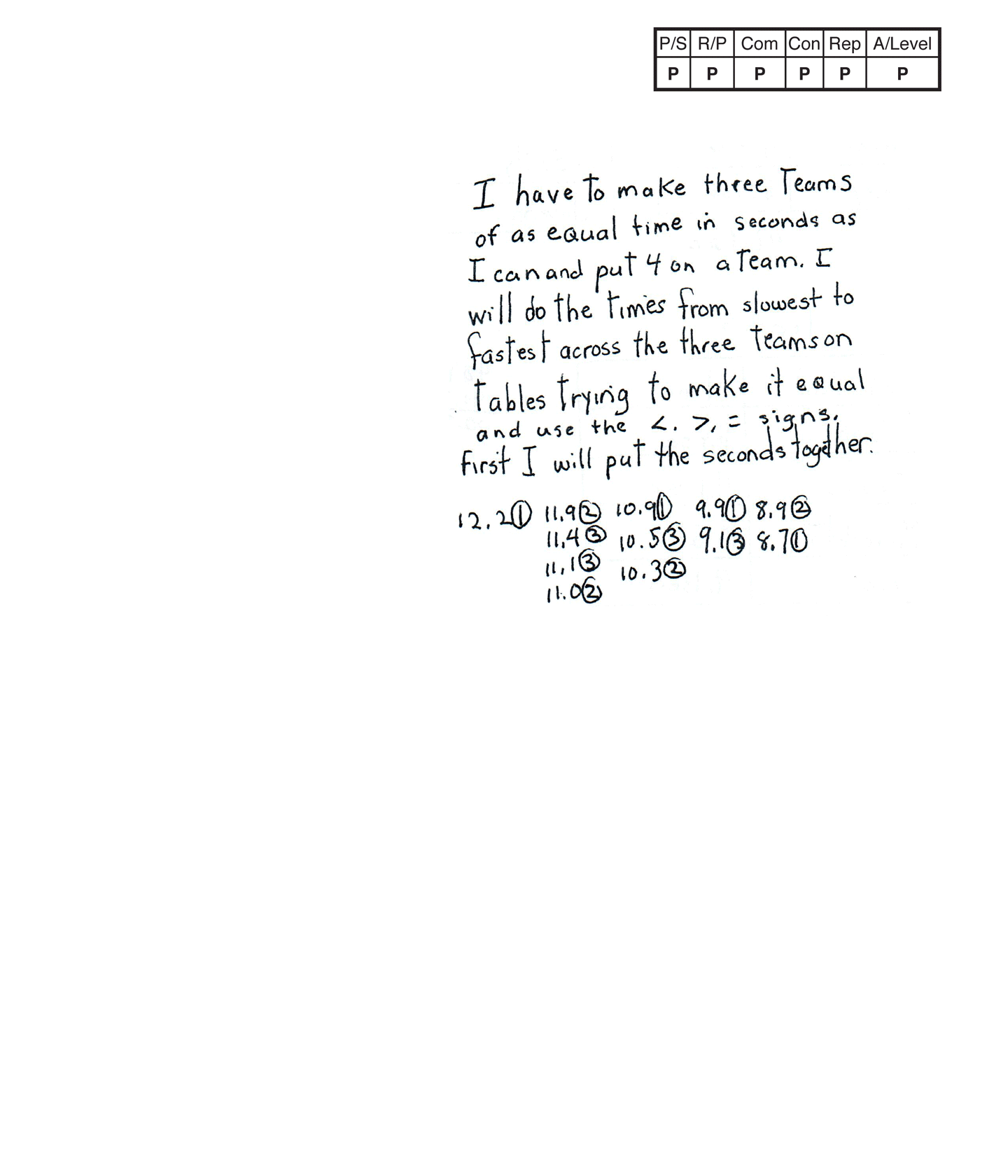,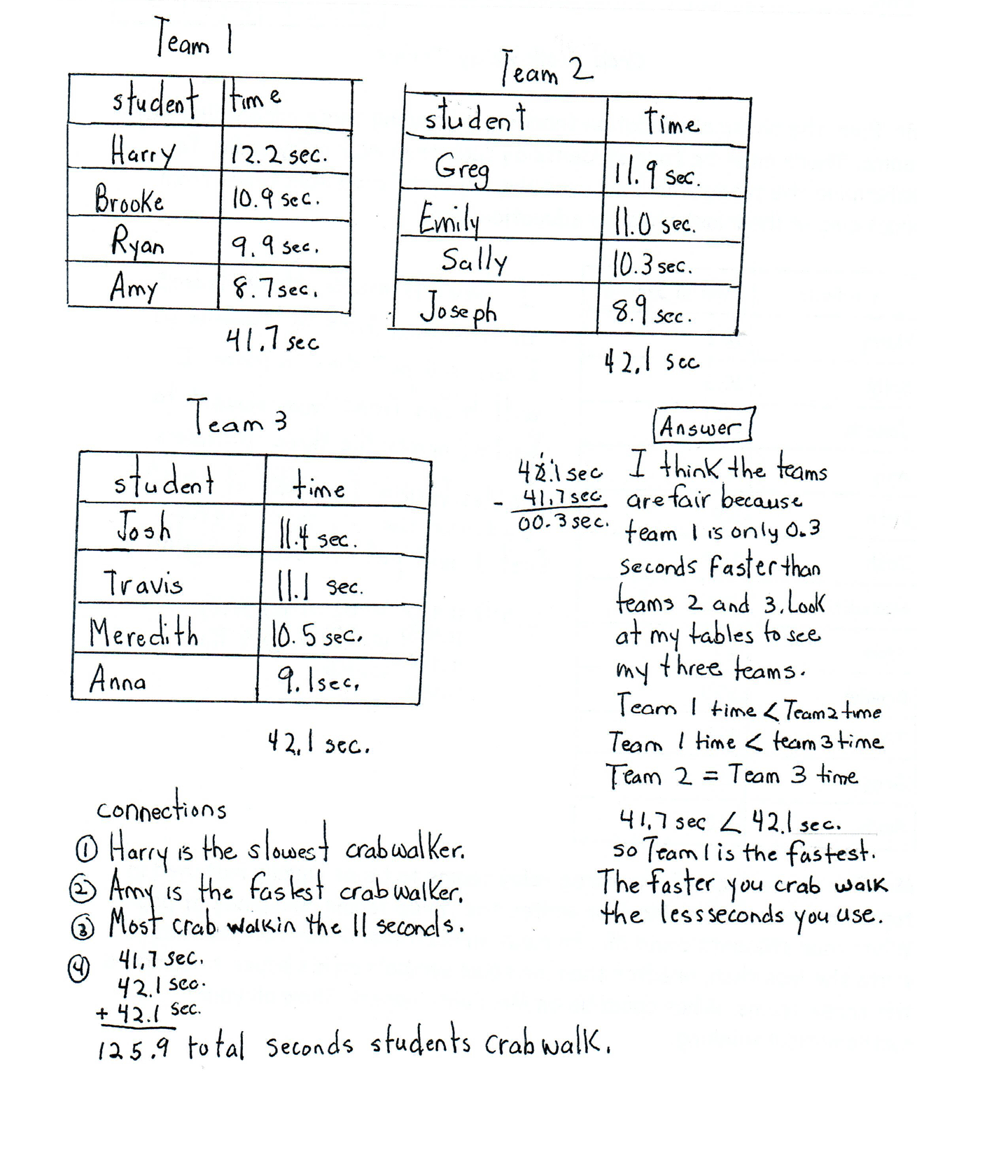This student meets the standard.

Scoring Rationale

Practitioner

The student's strategy of organizing the number of students in the 12-second, 11-second, nine-second, and eight-second range, creating three teams of four students, and calculating the total seconds per team to determine if they are equally matched works to solve the task. The student's answer, "I think the teams are fair because team 1 is only 0.4 seconds faster than teams 2 and 3. Look at my tables to see my three teams," is correct. The student correctly uses the less than, greater than, and equal signs to solve the second part of the task. The student correctly states, "Team 1 time < Team 2 time," "Team 1 time < Team 3 time," "Team 2 = Team 3 time," "41.7 < 42.1 sec. so Team 1 is the fastest."

Practitioner

The student demonstrates understanding that Mr. Fair requires three teams of four students that are as equally matched as possible. The student also shows correct reasoning in their use of the less than, greater than, or equal sign.

Practitioner

The student correctly uses the mathematical terms time(s), seconds (sec), equal from the task. The student also correctly uses the terms tables, fair, total. The student correctly uses the notation 12.2, 10.3, 8.9, 8.7, 9.9, 11.4, 10.5, 9.1, 10.9, 11.1, 11.9, 11.0 from the task. The student also correctly uses the notation 41.7, 42.1, 0.4, 125.9, <, >.

Practitioner

The student makes the mathematically relevant observations, "Harry is the slowest crab walker," "Amy is the fastest crab walker," "Most crab walk in the 11 seconds," "41.7 sec. + 42.1 sec. + 42.1 sec. = 125.9 total seconds students crab walk," and "The faster you crab walk the less seconds you use."

Practitioner

The student's three tables are appropriate to the task and accurate. Each table is correctly labeled, all entered data is correct, and includes the seconds unit.

Practitioner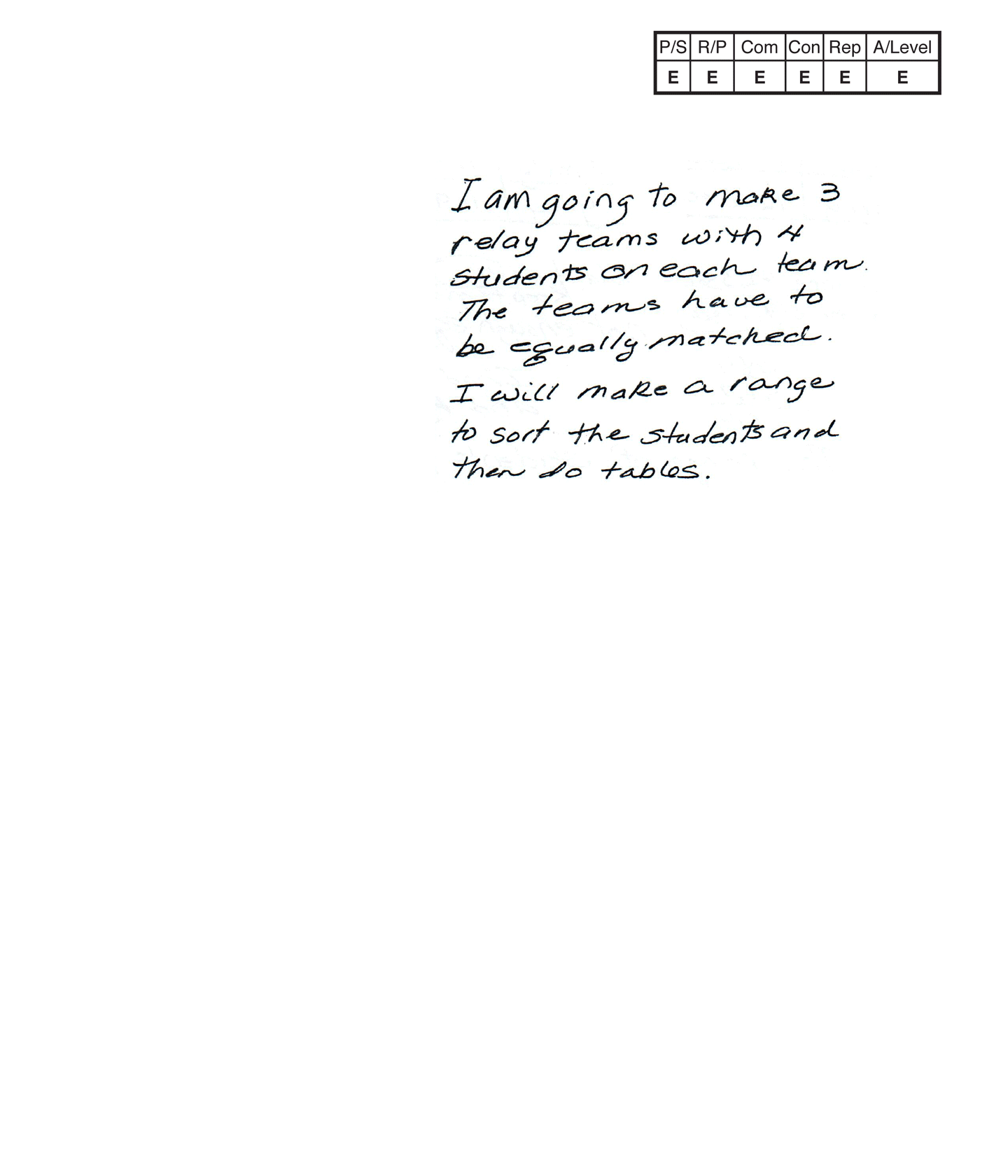,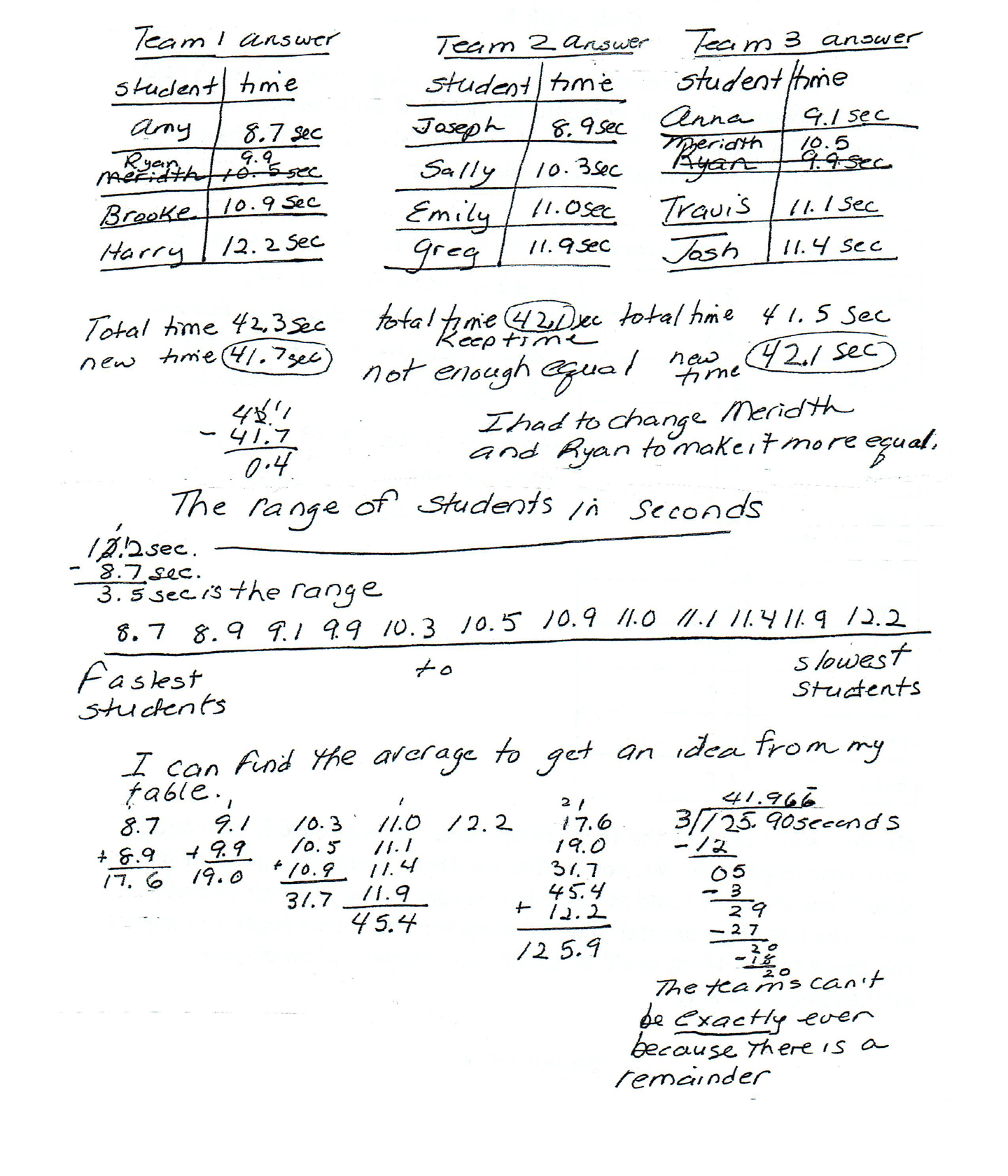,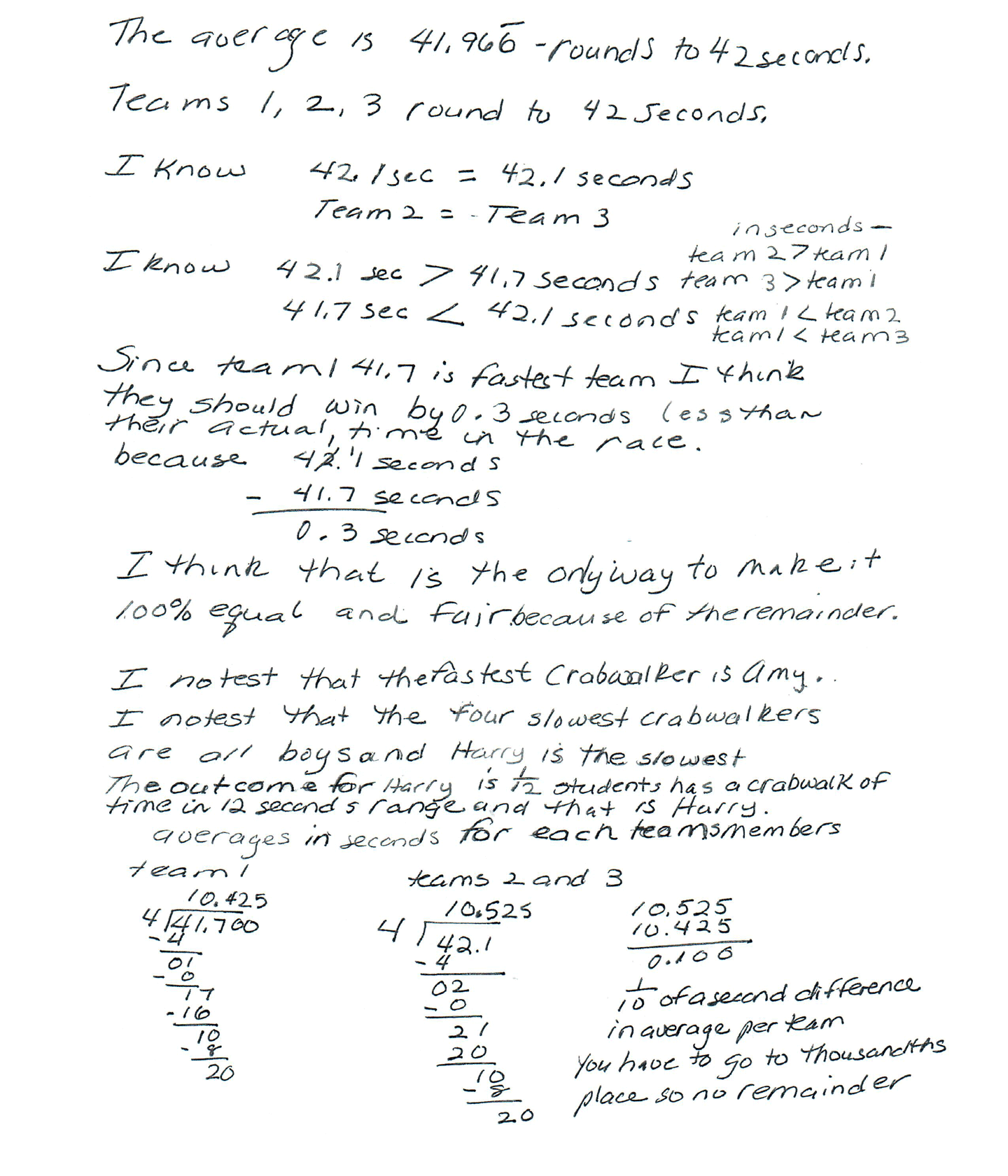This student exceeds the standard.

Scoring Rationale

Expert

The student's strategy of using tables to show "Team 1 answer, Team 2 answer, and Team 3 answer" for crab walk teams, works to solve the first part of the task. The student correctly uses the less than, greater than, and equal sign to compare the three teams to solve the second part of the task. The student's answer, "I know 42.1 = 42.1, I know 42.1 > 41.7 seconds, 41.7 < 42.1 seconds," and "in seconds-team 2 > team 1, team 3 > team 1, team 1 < team 2, team 1 < team 3," is correct. The student brings prior knowledge of range, average, percent, outcome to their solution.

Expert

The student demonstrates understanding that Mr. Fair requires three possible teams of four students that are as equally matched as possible. The student demonstrates understanding of correctly using the less than, greater than, and equal symbols to compare the three crab walk teams. The student brings the conceptual understanding of range and outcomes to their solution. The student applies the concept of average and percent to support their reasoning that when using Mr. Fair's data, you can not have three equally timed crab walk teams.

Expert

The student correctly uses the mathematical terms time, seconds (sec), equally, total from the task. The student also correctly uses the terms range, average, more than, fair, even, remainder, outcome, difference, per, thousandths place. The student correctly uses the notation 8.7, 8.9, 9.1, 9.9, 10.3, 10.5, 10.9, 11.0, 11.1, 11.4, 11.9, 12.2 from the task. The student also correctly uses the notation 3.5, 17.6, 19.0, 31.7, 45.4, 125.9, 41.966, 42.3, 42.1, 41.5, 41.7, 0.4, 1/12, 10.425, 10.525, 0.100, >, <, 100%.

Expert

The student makes the mathematically relevant Practitioner observations, "I notest that the fastest crabwalker is Amy," "I notest that the four slowest crabwalkers are all boys and Harry is the slowest." The student makes Expert connections by extending their solution to other mathematical concepts. The student states, "3.5 sec is the range." The student states, "I can find the average to get an idea from my table," and concludes, "The teams can't be exactly even because there is a remainder." The student justifies how adjusting the time would make the three teams equal. The student states, "Since team 1 41.7 is fastest team I think they should win by 0.4 seconds less than their actual time in the race," "I think that is the only way to make it 100% equal and fair because of the remainder." The student extends their thinking to finding outcomes. The student states, "The outcome for Harry is 1/12 students has a crabwalk of time in 12 seconds range and that is Harry." The student finds the "averages in seconds for each teams members." The student finds 10.425 for team one and 10.525 for teams two and three. The student states, "1/10 of a second difference in average per team. You have to go to thousandths place so no remainder."

Expert

The student's three tables are appropriate to the task and accurate. Each tabled is titled, the columns are correctly labeled and all entered data is correct. The student uses the data on their tables to extend their thinking to range, average, and percent.